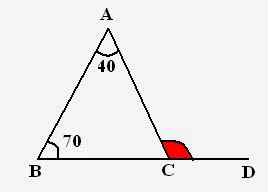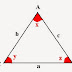Geometry is one of the most important topics of Quantitative Aptitude section of SSC CGL exam. It includes various concepts related to lines, angles, triangles, circles, polygons and so on. So, today I will just discuss concept and properties of triangle. Always remember that in Geometry, You need a very basic understanding. Cramming is not gonna help you anywhere.

## Triangles

Triangle = Figure with three sides.
Study the following triangle:
A,B,C to points.
a, b, c to sides.
x, y, z to angles.Perimeter of triangle = a + b + c
Remember that, Sum of all the angles is always 180^o

i.e. x + y + z = 180^o

### Classification of Triangles

Basically there are three types of triangles excluding right angle triangle. Let me tell you how they vary from each other.
• Scalene Triangle
• Isosceles Triangle
• Equilateral Triangle
Scalene Triangle: No side of triangle is equal.
Isosceles Triangle: Two sides of triangle are equal.
Equilateral Triangle: All sides of triangle are equal.

 Scalene Isosceles Equilateral Definition a ne b ne c ane b = c a = b = c Area A (b/4) sqrt{4a^2 – b^2} (3)^(1/2)(a^2)/4 Height - sqrt{(4a^2-b^2) /2} (3)^(1/2)a/2

A = {s(s-a)(s-b)(s-c)}^{1/2}
where, s = (a+ b+ c)/2

### Properties of external angles of Triangle:

1. Sum of all exterior angles is 360^o

Study the following set of triangles and their exterior angles,a, b, c to Interior angles.
p, q, r and s, t, u to Exterior angles.

So, sum of exterior angles = 360^o
i.e. p + q + r = 360^o and
s + t + u = 360^o

2. Next property of exterior angle which is important in paper point of view:

External angle = Sum of two internal angles.

For example: In above figures,
r = a + b
q = a + c
s = b + c and so on.

## Right angle Triangle

Following triangle is a right angle triangle i.e. a triangle with one out of three 90^o angle.### Area of right angle triangle

Area = 1/2 times Base times Perpendicular

### Example with Solution

Example: In following figure, CE is perpendicular to AB, angle ACE = 20^o and angle ABD = 50^o. Find angle BDA:Solution: To Find: angle BDA

For this what we need --- angle BAD
Because, Sum of all angles = 180 ^o

Consider, triangle ECA,

CEA + EAC + ACE = 180^o
i.e. 90^o + 20^o + EAC = 180^o
Therefore, EAC = 70^o

Now, come to triangle ABD,

ABD + BDA + BAD = 180^o
70^o + 50^o + BAD = 180^o
Therefore, BAD = 60^o

Example: In given figure. BC is produced to D and angle BAC = 40^o and angle ABC = 70^o. Find angle ACD:Solution: In above figure, ACD is an exterior angle, and according to property,
Exterior angle = Sum of interior angles
Therefore, ACD = 70^o + 40^o
=110^o

This is not the end of this chapter. These are just the basics. In next session, I will discuss some important results, properties (congruency, similarity) and much more.
Always remember, Geometry needs practice and time.

Also check:
Important Questions of Geometry for SSC CGL

Geometry -concepts and properties for SSC CGL Tier-IReviewed by Jasleen Behl on Wednesday, February 12, 2014 Rating: 5

1.When new session is going to be held. ..?

•This comment has been removed by the author.

•I have already discussed which i have mentioned.... Following is the link of the topic:
https://plainmath.net/2014/02/geometry-part-2-for-ssc-cgl-tier-i.html
https://plainmath.net/2014/03/gemetrypart-3-circles-and-their.html
Thnx..

2.Gud gab

3.Chetanreddy KampaOctober 9, 2015 at 3:15 AM

Gud gab

4.thanks

I will try to respond asap